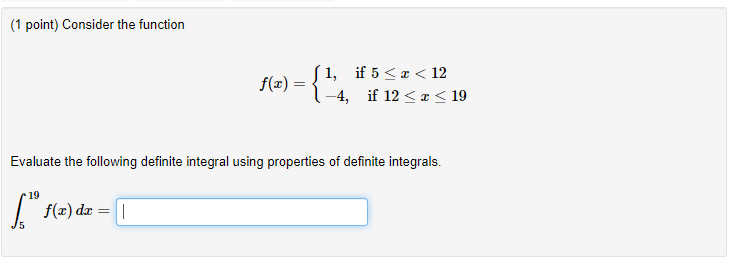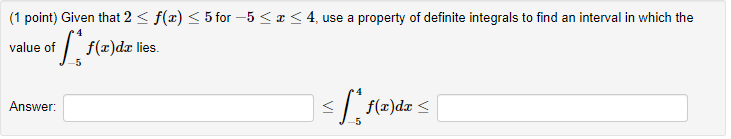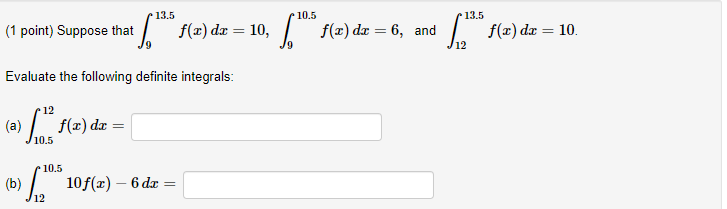# Question A)B)C) (1 point) Consider the function f(x) = 1, if 5 <<<12 4, if 12 << < 19 Evaluate the following definite integral using properties of definite integrals. 6" f(x) dx = (1 point) Given that 2 <f(x) < 5 for –5 <<4, use a property of definite integrals to find an interval in which the value of f(x)dar lies of [flada Answer: < *Lflada < 13.5 10.5 13.5 (1 point) Suppose that | ** f(a) da = 10, 4, *** $(2) de = 6, and f(x) dx = 10 /9 Evaluate the following definite integrals: 12 ( fx = 10.5 a) "*$(x) de 5 % * 10f(a) – 6de = 10.5 (b) ) 6 = .A)B)C)Transcribed Image Text: (1 point) Consider the function f(x) = 1, if 5 <<<12 4, if 12 << < 19 Evaluate the following definite integral using properties of definite integrals. 6" f(x) dx = (1 point) Given that 2
More
Transcribed Image Text: (1 point) Consider the function f(x) = 1, if 5 <<<12 4, if 12 << < 19 Evaluate the following definite integral using properties of definite integrals. 6" f(x) dx = (1 point) Given that 2